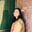Related Tags

c

# What is cpowl() in C?Umme Ammara

The cpowl function is a C library function that raises a complex number to a complex power.

The following illustration shows the functionality of the cpowl function:

To use the cpowl function, we need to include the complex.h header file, as shown below:

#include <complex.h>


## Syntax

The cpowl function takes two arguments and returns the complex power. It is declared as follows:

## Parameters

The cpowl function takes two arguments of the long double complex type.

• The first parameter is the number we want to raise.

• The second parameter is the power with which we want to raise the number.

## Return Value

The cpowl function computes and returns the power in the long double complex type.

## Example

The code below shows the use of cpowl function in C:

#include <stdio.h>
#include <complex.h>

int main() {

//Declare x and y
long double complex x = -1.5 + I*1.5;
long double complex y = 3.0;

//Call cpowl i.e x^y
long double complex ans = cpowl (x,y);

//Display the result
printf ("(1+2i)^3 = %f%+fi\n", creal(ans), cimag(ans) );

return 0;
}

RELATED TAGS

c

CONTRIBUTORUmme Ammara